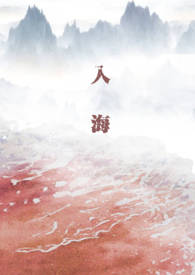# 入海

• 一.认路
• 二.仇人
• 三.初遇
• 四.女孩
• 五.该死
• 六.毒
• 七.对不起
• 八.你去哪里了
• 九.欠你的
• 十.配合一下
• 十一.入门
• 十二.说谎
• 十三.不必忍
• 十四.筑基
• 十五.愁肠百转
• 十六.最后一次
• 十七.水灵根
• 十八.病
• 十九.蓬莱岛
• 二十.面善
• 二十一.抓住你了
• 二十二.地宫
• 二十三.楚春寒
• 二十四.找到
• 二十五.混乱
• 二十六.冷战
• 二十七.浑水摸鱼
• 二十八.道歉
• 二十九.喜欢
• 三十.谎言
• 三十一.亲吻
• 三十二.睡觉
• 三十三.约定
• 三十四.原谅
• 三十五.浮游
• 三十六.预言
• 三十七.舍不得
• 三十八.回家
• 三十九.别看
• 四十.如今
• 四十一.冲突
• 四十二.封丹
• 番外珍珠
• 四十三.公主殿下
• 四十四.契约
• 四十五.怎么办
• 四十六.不要
• 四十七.说话算数
• 四十八.花
• 四十九.坦诚
• 五十.好久不见
• 五十一.眼熟
• 五十二.七百年
• 五十三.别摸了
• 五十四.讨价还价
• 五十五.朋友
• 五十六.冤冤相报
• 五十七.报答
• 五十八.生气
• 五十九.慢慢来
• 六十.魇兽
• 六十一.噩梦
• 六十二.对不起
• 六十三.亲我一下
• 六十四.安宁殿
• 六十五.真相
• 六十六.子桑国
• 六十七.一路顺风
• 六十八.画
• 六十九.花样
• 七十.小肚鸡肠
• 七十一.偶遇
• 七十二.故地
• 现代AU
• 七十三.秦秧
• 吸血鬼AU—1
• 吸血鬼AU—2
• 吸血鬼AU—3
• 七十四.不是鱼
• 七十五.契
• 七十六.双灵根
• 七十七.不反抗
• 七十八.小狗
• 七十九.不好
• 八十.忍住
• 八十一.生死契
• 八十二.悉数奉还
• 八十三.对不起
• 八十四.不值
• 八十五.狐妖
• 八十六.夭竹
• 八十七.吃味
• 八十八.化形
• 八十九.要你
• 九十.难受
• 九十一.真乖
• 九十二.积德
• 九十三.事端
• 九十四.怎么回事
• 九十五.问好
• 九十六.保护
• 九十七.姐姐
• 九十八.别怕
• 九十九.耽误
• 一百.猫妖
• 一百零一.堕魔
• 一百零二.阿姐
• 一百零三.地头蛇
• 一百零四.相救
• 一百零五.福兆
• 一百零六.报仇
• 一百零七.旧忆
• 一百零八.废物
• 一百零九.说谎
• 一百一十.梦碎
• 一百一十一.灯灭
• 一百一十二.身死
• 一百一十三.杀
• 一百一十四.魔功
• 吸血鬼AU—4
• 吸血鬼AU—5
• 一百一十五.管我
• 一百一十六.因果
• 一百一十七.等我
• 一百一十八.母亲
• 一百一十九.早去早回
• 一百二十.重逢sёxiaòshu.c ò м
• 一百二十一.狡猾
• 一百二十二.及时行乐
• 一百二十三.好姑娘
• 一百二十四.自己来
• 一百二十五.乖
• 一百二十六.玉人 53℃e.℃o㎡
• 一百二十七.不归墟
• 一百二十八.福气
• 一百二十九.可怕
• 一百三十.抢劫 нá𝔦𝓽á𝓃𝑔𝓌ô.𝒸ô𝓂
• 一百三十一.好看
• 一百三十二.着迷 ρò1８vs.čòм
• 一百三十三.夸奖
• 一百三十四.忘了 р𝑜18α𝖚.𝒸𝑜м
• 一百三十五.出错
• 一百三十六.冲动
• 一百三十七.模样
• 中秋番外——练剑日记
• 一百三十八.故人
• 一百三十九.恶心
• 一百四十.帮忙
• 一百四十一.针眼
• 一百四十二.家
• 一百四十三.过分
• 一百四十四.信任
• 一百四十五.跟踪 𝓷ⅰнō𝓷𝓰𝓰𝔢.čōⅿ
• 一百四十六.生气
• 吸血鬼AU—6
• 吸血鬼AU—7
• 一百四十七.葬身之地
• 一百四十八.废人 𝔭𝖔18𝖈в.𝓬𝖔𝓂
• 一百四十九.逃
• 一百五十.复仇
• 一百五十一.请见
• 一百五十二.虚假 ρ𝑜18α𝖚.𝒸𝑜𝓂
• 一百五十三.试探
• 一百五十四.西南 ρǒ18čb.čǒm
• 一百五十五.不能
• 一百五十六.小月
• 一百五十七.带话
• 一百五十八.演
• 一百五十九.可爱
• 一百六十.刺激 ρō18čκ.čō㎡
• 一百六十一.汪
• 一百六十二.绑架
• 一百六十三.母女 ý𝓊ｚhaǐw𝓊h.𝓍ýz
• 一百六十四.雪
• 一百六十五.夜话
• 一百六十六.相见
• 一百六十七.不信
• 一百六十八.一场空
• 一百六十九.重逢 hei yeshuku.com
• 一百七十.阴谋
• 姬婞番外（1）
• 姬婞番外（2）
• 姬婞番外（终）
• 一百七十一.守住
• 一百七十二.倒打一耙
• 一百七十三.下场
• 一百七十四.好久不见
• 一百七十五.暴露
• 一百七十六.是不是
• 一百七十七.大补 iyuzhai wu.xy z
• 一百七十八.散去